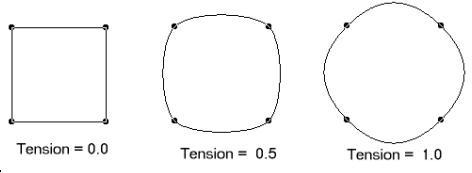﻿ Tension Property (AnnCurveObject) | Leadtools.Annotations.Engine | HTML5 JavaScript Help
In This Topic ▼

# tension Property

Summary

Gets or sets a value that specifies the amount that this AnnCurveObject curve bends between control points.

Syntax
TypeScript
JavaScript
````Object.defineProperty(AnnCurveObject.prototype, 'tension', `
`	get: function(), `
`	set: function(value) `
`) ````
``tension: number; ``

#### Property Value

A value that specifies the amount that this AnnCurveObject curve bends between control points.

Remarks

Valid values are between 0.0 and 1.0, where 0.0 indicates straight lines, and 1.0 indicates lines with the highest curvature. A typical value is 0.5. These values for Tension for a Closed Curve object are shown in the following bitmap:Requirements